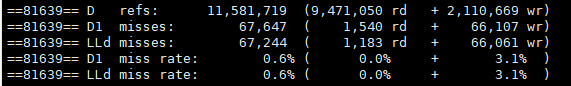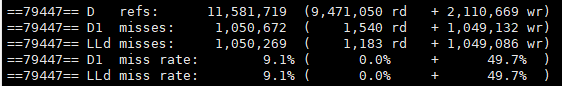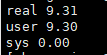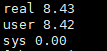## Clean Code in the Eyes of Compiler Engineers (1): Loop Interchange

2022-08-02loop interchangeBiSheng Compiler

Abstract: During C/C++ code compilation, the compiler translates source code into an instruction sequence that can be identified by CPUs and then generates the executable code, on which the running efficiency of the final code depends. In most cases, the source code determines the extent to which a program can be optimized by the compiler. Even minor changes to the source code can exert a significant impact on the running efficiency of the code generated by the compiler. Therefore, source code optimization can help the compiler generate more efficient executable code to some extent.

This blog uses loop interchange as an example to describe how to write code to obtain better performance.

## 1. Basic Concepts of Loop Interchange #

### 1.1 Locality of Reference #

In computer science, locality of reference refers to that an application tends to access relatively close values in the memory. Locality is a type of predictable behavior that occurs in computer systems. Systems that exhibit strong locality of reference are great candidates for performance optimization. There are three basic types of reference locality: temporal locality, spatial locality, and sequential locality.

Loop interchange described in this blog mainly uses spatial locality. Spatial locality refers to that recently referenced memory locations and their surrounding locations tend to be used again. This is common in loops. For example, in an array, if the third element is used in the previous loop, it is very likely that the fourth element will be used in the current loop. If the fourth element is indeed used in the current loop, the cache data prefetched in the previous iteration is hit.

Therefore, array loop operations can utilize the spatial locality feature to ensure that accesses to array elements in two adjacent loops are closer in the memory. That is, the smaller the stride when the elements in an array are accessed cyclically, the corresponding performance may be better. That is, a smaller stride can lead to better performance to cyclically access array elements.

Next, let's see how arrays are stored in the memory.

### 1.2 Row-major Order and Column-major Order #

Row-major order and column-major order are two methods for storing multidimensional arrays in linear storage. The elements of an array are consecutive in the memory. Row-major order indicates that the consecutive elements of a row are adjacent to each other in the memory, and column-major order indicates that the consecutive elements of a column are adjacent to each other. See the following figures.While the terms allude to the rows and columns of a two-dimensional array, row-major and column-major orders can be generalized to arrays of any dimension.

But which of the preceding methods is used to store arrays in C/C++?

The following example uses the cachegrind tool to compare the cache loss rates of CPUs when C uses two different access methods.

Access by row:

``````#include <stdio.h>

int main(){
size_t i,j;
const size_t dim = 1024 ;
int matrix [dim][dim];

for (i=0;i< dim; i++)
for (j=0;j <dim;j++)
matrix[i][j]= i*j;

return 0;
}
``````Access by column:

``````#include <stdio.h>

int main(){

size_t i,j;

const size_t dim = 1024 ;

int matrix [dim][dim];

for (i=0;i< dim; i++){
for (j=0;j <dim;j++){
matrix[j][i]= i*j;
}
}
return 0;
}
``````According to the comparison between cache loss rates of the two access methods of the same array in the preceding C code, it can be noted that in C/C++ code, arrays are stored in row-major order. That is, if `a[i][j]` is accessed in the previous loop, the access to `a[i][j+1]` will hit the cache. In this way, the main memory will not be accessed. Because access to the cache is faster, following the storage order of a corresponding programming language to hit the cache can implement optimization.

Other common programming languages, such as Fortran and MATLAB, use the default column-major order.

### 1.3 Loop Interchange #

Based on the row-major order of C/C++, loop interchange uses the feature that the system tends to access values close to each other in the memory to exchange the execution order of two loops in a nested loop to increase the overall spatial locality of the code. In addition, it can enable other important code conversions. For example, loop reordering is the optimization of loop interchange that reorders two and more loops. In LLVM, to use loop interchange, enable the `-mllvm -enable-loopinterchange` option.

## 2. Optimization Examples #

### 2.1 Basic Scenarios #

The following is an example of a matrix operation.

Original code:

``````for(int i = 0; i < 2048; i++) {
for(int j = 0; j < 1024; j++) {
for(int k = 0; k < 1024; k++)  {
C[i * 1024 + j] += A[i * 1024 + k] * B[k * 1024 + j];
}
}
}
``````

Code after loop interchange is performed on the `j and k` loops:

``````for(int i = 0; i < 2048; i++) {
for(int k = 0; k < 1024; k++) {
for(int j = 0; j < 1024; j++)  {
C[i * 1024 + j] += A[i * 1024 + k] * B[k * 1024 + j];
}
}
}
``````

It is found that in the original code, each time the innermost `k` loop is iterated, the data accessed by `C` remains unchanged, the access stride for `A` is always 1, and there is a high probability that the cache is hit. However, because the access stride for `B` each time is 1024, the cache misses almost every time. After loop interchange is performed, the `j` loop is the innermost loop. Each time the `j` loop is iterated, the data accessed by `A` remains unchanged; and because the access stride for `C` and `B` is always 1, there is a high probability that the cache is hit. In this way, the cache hit ratio is greatly increased.

Does the cache hit ratio really increase in actual applications and how does the performance change?

Original code:

``````\$ time -p ./a.out
````````````\$ sudo perf stat -r 3 -e cache-misses,cache-references,L1-dcache-load-misses,L1-dcache-loads ./a.out
``````Result after Loop Interchange:

``````\$ time -p ./a.out
````````````\$ sudo perf stat -r 3 -e cache-misses,cache-references,L1-dcache-load-misses,L1-dcache-loads ./a.out
``````Comparison:

The number of L1-dcache-loads is almost the same because the total amount of data to be accessed is the same.

The ratio of L1-dcache-load-misses to L1-dcache-loads is reduced by nearly 10 times after loop interchange.

In addition, the performance is improved by 9.5%.

### 2.2 Special Scenarios #

In actual applications, not all scenarios are like the loop interchange scenario described in section 2.1.

``````for ( int i = 0; i < N; ++ i )
{
if( I[i] != 1 ) continue;

for ( int m = 0; m < M; ++ m )
{
Res2 = res[m][i] * res[m][i];
norm[m] += Res2;
}
}
``````

For example, in the preceding scenario, if N is an ultra-large array, loop interchange can bring great benefits theoretically. However, an if branch judgment is added between the two loops, hindering the implementation of loop interchange.

In this case, you can remove the branch judgment logic in the middle to ensure that loop interchange can enable the array `res` to be accessed in the continuous memory. After the loop interchange is performed on the two loops, you can roll back the branch judgment logic.

``````for ( int m = 0; m < M; ++ m )
{
for ( int i = 0; i < N; ++ i)
{

Res2 = res[m][i] * res[m][i];
norm[m] += Res2;

if( I[i] != 1 )  // Add logic to ensure source code semantics.
{
norm[m] -= Res2;
continue;
}
}
}
``````

You also need to consider whether the cost is worthwhile for such source code modification. If the if branch is entered frequently, the cost caused by subsequent rollback is high. In this case, you may need to reconsider whether the loop interchange is worthwhile. Conversely, if the branch entry frequency is low, the implementation of loop interchange can bring considerable benefits.

## 3. Contribution of the BiSheng Compiler to the LLVM Community #

The BiSheng Compiler team has made great contributions to the loop interchange pass in the LLVM community. The team enhanced the loop interchange pass in terms of legality and profitability, and extended the scenarios supported by the pass. In terms of loop interchange, the team has provided more than 20 major patches for the community in the past two years, including loop interchange and related analysis and optimization enhancements such as dependence analysis, loop cache analysis, and delinearization. The following are some examples:

The two patches extend the application of loop interchange to scenarios where the inner or outer loop contains more than one induction variable.

``````for (c = 0, e = 1; c + e < 150; c++, e++) {
d = 5;
for (; d; d--)
a |= b[d + e][c + 9];
}
}
``````

The two patches extend the application of loop interchange to scenarios where reduction calculation of the floating-point type is supported.

``````double matrix[dim][dim];
for (j=0;j< dim; j++)
for (i=0;i <dim;i++)
matrix[i][j] += 1.0;
``````

D120386 [LoopInterchange] Try to achieve the most optimal access pattern after interchange (https://reviews.llvm.org/D120386)

This patch enhances the interchange capability so that the compiler can permute the loop body to the globally optimal loop sequence.

``````void f(int e, int f) {
for (int a = 0; a < 100; a++) {
for (int b = 0; b < 100; b++) {
for (int c = 0; c < 100; c++) {
f[c][b][a] = e[c][b][a];
}
}
}
``````

=>

``````void f(int e, int f) {
for (int c = 0;  c < 100; c++) {
for (int b = 0; b < 100; b++) {
for (int a = 0; a < 100; a++) {
f[c][b][a] = e[c][b][a];
}
}
}
}
``````

D124926 [LoopInterchange] New cost model for loop interchange (https://reviews.llvm.org/D124926)

This patch provides a new and more powerful cost model for loop interchange, which can accurately determine the loop interchange profitability.

In addition, the team has contributed a large number of bugfix patches to the community.

• D102300 [LoopInterchange] Check lcssa phis in the inner latch in scenarios of multi-level nested loops

• D101305 [LoopInterchange] Fix legality for triangular loops

• D100792 [LoopInterchange] Handle lcssa PHIs with multiple predecessors

• D98263 [LoopInterchange] fix tightlyNested() in LoopInterchange legality

• D98475 [LoopInterchange] Fix transformation bugs in loop interchange

• D102743 [LoopInterchange] Handle movement of reduction phis appropriately during transformation (pr43326 && pr48212)

• D128877 [LoopCacheAnalysis] Fix a type mismatch bug in cost calculation

The following are enhancements to other functions:

• D115238 [LoopInterchange] Remove a limitation in legality

• D118102 [LoopInterchange] Detect output dependency of a store instruction with itself

• D123559 [DA] Refactor with a better API

• D122776 [NFC][LoopCacheAnalysis] Add a motivating test case for improved loop cache analysis cost calculation

• D124984 [NFC][LoopCacheAnalysis] Update test cases to make sure the outputs follow the right order

• D124725 [NFC][LoopCacheAnalysis] Use stable_sort() to avoid non-deterministic print output

• D127342 [TargetTransformInfo] Added an option for the cache line size

• D124745 [Delinearization] Refactoring of fixed-size array delinearization

• D122857 [LoopCacheAnalysis] Enable delinearization of fixed sized array

## Conclusion #

To use loop interchange for optimization as much as possible, ensure that the access stride of arrays or number sequences between iterations is as small as possible when writing C/C++ code. The closer the stride is to 1, the higher the spatial locality is, the higher the natural cache hit ratio is, and the more ideal benefits can be obtained in performance. In addition, the storage method of C/C++ is row-major order. Therefore, when accessing a multidimensional array, ensure that the inner loop must be Column to obtain a smaller stride.